S k i l l
i n
A R I T H M E T I C

Lesson 12

# DIVIDING DECIMALS

Dividing decimals by the traditional written method no longer serves any practical or educational purpose. The educational point on which that method is based is the following, and we have addressed it:

If we multiply the dividend and divisor by the same number,
the quotient will not change.

(Lesson 10; see Problems 17, 18.)

We have already seen certain cases of dividing decimals. In Lesson 10 we used the division bar, which is now the most useful form to indicate division. In Lesson 11, however, we did problems with the traditional division box. For the sake of those students still required to learn division of decimals in that way, there remains only the case in which the divisor is a decimal.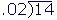But we have seen that the divisor must be a whole number. If it is not, then we will multiply it so that it becomes one.

 When the divisor is a decimal, what must we do?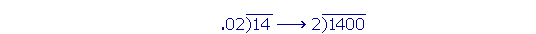1) Multiply the divisor by 10, or 100, or 1000, etc., according to the number of decimal places, so that it becomes a whole number. 2) Multiply the dividend by the same power of 10.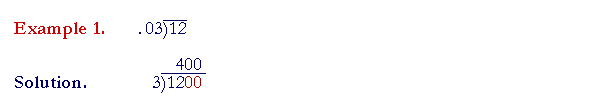Multiply the divisor by 100 so that it becomes the whole number 3.  Compensate by multiplying 12 by 100 also; add on two 0's.  (Lesson 3, Question 1.)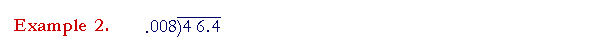Make .008 into the whole number 8 by multiplying it by 1000.  Multiply the dividend also by 1000: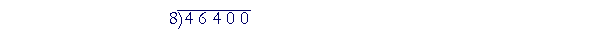Move the decimal point three places right.  (Lesson 3, Question 2.)

Notice that the dividend becomes a whole number.  There is no decimal point.  Therefore there will be no decimal point in the quotient: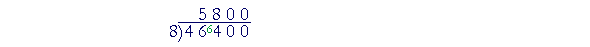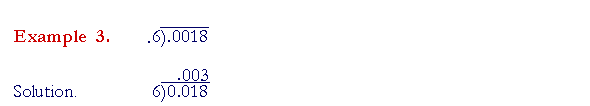Multply both numbers by 10.  The decimal point then goes directly above.

At this point, please "turn" the page and do some Problems.

or

Continue on to the next Lesson.

1st Lesson on Division

www.proyectosalonhogar.com